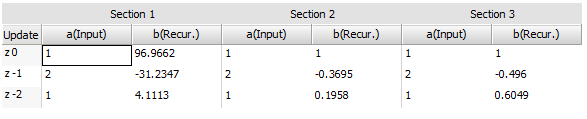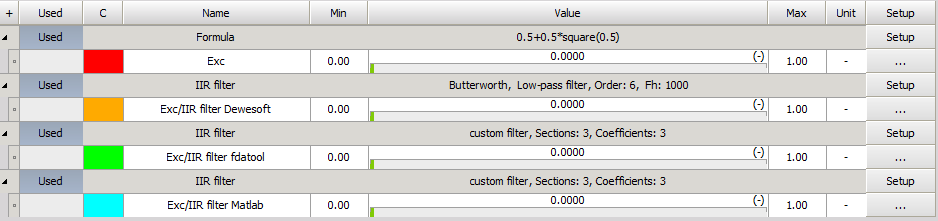In Dewesoft we offer the possibility to import custom IIR filters created in other environments such as Matlab.

When creating filter in Matlab the filter coefficients are presented in SOS matrix form without considering the gain, which is calculated and presented separately. In Dewesoft, you can’t enter the gain separately so you have to include it in the filter coefficients. All you have to do is scale the coefficients the right way. Let's see how to correctly scale the factors on the following examples.

In both cases a 6th order lowpass Butterworth IIR filter is created, with the cut-off frequency at 1000 Hz at 5000 Hz sample rate.

Example #1: Creating filter with Filter Design & Analysis Tool ("fdatool")
Enter the settings for the chosen filter in the "fdatool", where 'Fs' presents sample rate and 'Fc' the cut-off frequency:To process the SOS matrix and gain vector, the data need to be exported in to the workspace.We get the following results:Each row of the SOS matrix represents different section. The first three (1:3) values in the row represent the “a(input)” coefficients. The 4:6 values represent the “b(Recur.)" coefficients, which have to be scaled/recalculated. The values have to be divided with the correct gain factor. For the calculation you can use the following equation:

SOS(n,4:6)/G(n)

After the recalculation we get the following coefficients:Be careful how you import the values in Dewesoft as the values in the matrices are differently arranged.Example #2: Creating filter in Matlab
To create zeros(z), poles(p) and coefficient(k) of the filter the following function is used:

[z,p,k]=butter(n,Wn,ftype)

The 'n' represents the order of the filter, 'Wn' represents the cutoff frequency dependent of sample rate (Wn = Fc/Fs/2) and 'ftype' represents the type of the filter ( low, high, bandpass, stop). In our case we use the following settings:

[z,p,k]=butter(6, 1000/2500, low)

From the z, p and k values the SOS matrix and gain are calculated with the following function:

[sos,g]=zp2sos(z,p,k)In this case the gain is a scalar and not a vector as in the previous example, but we can simply create a vector as:

g = [g;1;1]

and do the same procedure for the scaling/recalculating of the coefficients as before with the following function:

SOS(n,4:6)/G(n)The coefficients are slightly different as in the first example, but are in the same ratio which is important.Example #3: Compare filters from Example #1, Example #2 and filter created in Dewesoft.

We create a simple setup file with simulated Excitation signal (Exc) and three filtered channels using differently created filters with the same characteristics:We add the channels to the Modal test using Shaker as the Test method:We can see that all three filters gave us the same response, from which we can be sure that the filters are the same: